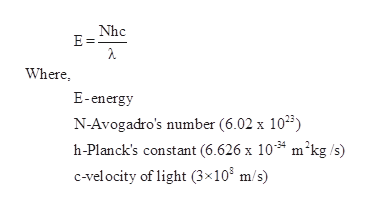# A particular sample of radiation has an energy content of 1.09x10^-3 J of energy. Calculate the number of moles of photons in this sample when the wavelegth of the light is 640nm.

Question
12 views

A particular sample of radiation has an energy content of 1.09x10^-3 J of energy. Calculate the number of moles of photons in this sample when the wavelegth of the light is 640nm.

check_circle

Step 1

Energy and number of moles of photons are related as,help_outlineImage TranscriptioncloseNhc E = Where E-energy N-Avogadro's number (6.02 x 1035) h-Planck's constant (6.626 x 10* m2kg /s) C-vel ocity of light (3x103 m/s) fullscreen
Step 2

The given vales are substitute in above equatio...

### Want to see the full answer?

See Solution

#### Want to see this answer and more?

Solutions are written by subject experts who are available 24/7. Questions are typically answered within 1 hour.*

See Solution
*Response times may vary by subject and question.
Tagged in

### Chemistry